# 【光学】基于matlab实现圆孔的菲涅尔衍射仿真

+关注继续查看

## 1 简介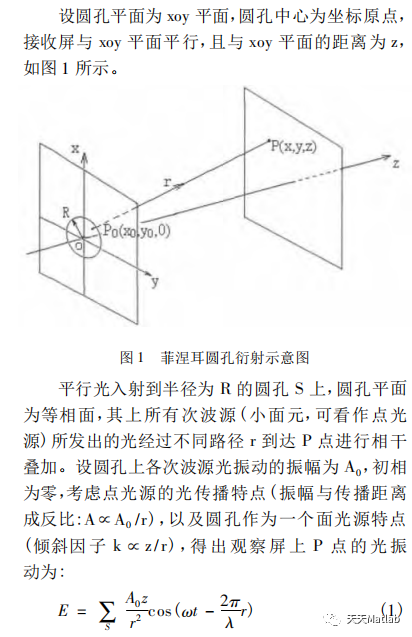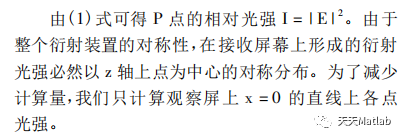## 2 部分代码

%圆孔衍射 菲涅尔clearlamda=633*10^(-6);k=2*pi/lamda;%?¨??p=1;z=100;fu=sqrt(-1);d=0.1;[X,Y]=meshgrid(-p:0.005:p,-p:0.005:p);for i=1:size(X,1)    for j=1:size(Y,1)        if X(i,j).^2+Y(i,j).^2<=(d/2)^2            A(i,j)=1;        else            A(i,j)=0;        end     endendendfigure(1)subplot(2,2,1);imshow(A,[])subplot(2,2,2);imshow(aabss,[])colormap(gray);subplot(2,2,3);[i,j]=size(aabss);plot(x,B)subplot(2,2,4);surfl(aabss)shading interpcolormap(gray)axis off

## 3 仿真结果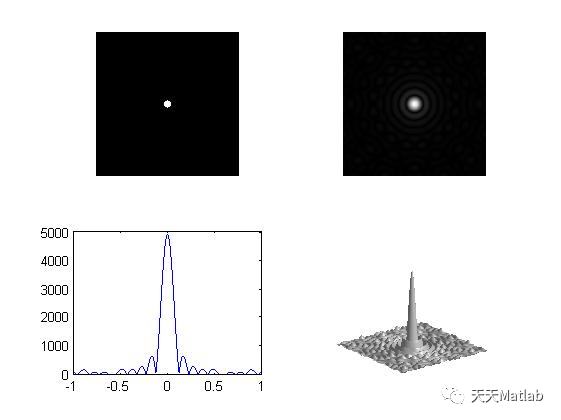## 4 参考文献

陈丽娜, 陆霁, 朱伟玲. 基于MATLAB对菲涅耳圆孔衍射的模拟[J]. 实验室科学, 2014, 17(4):4.

### 博主简介：擅长智能优化算法、神经网络预测、信号处理、元胞自动机、图像处理、路径规划、无人机等多种领域的Matlab仿真，相关matlab代码问题可私信交流。

#### 部分理论引用网络文献，若有侵权联系博主删除。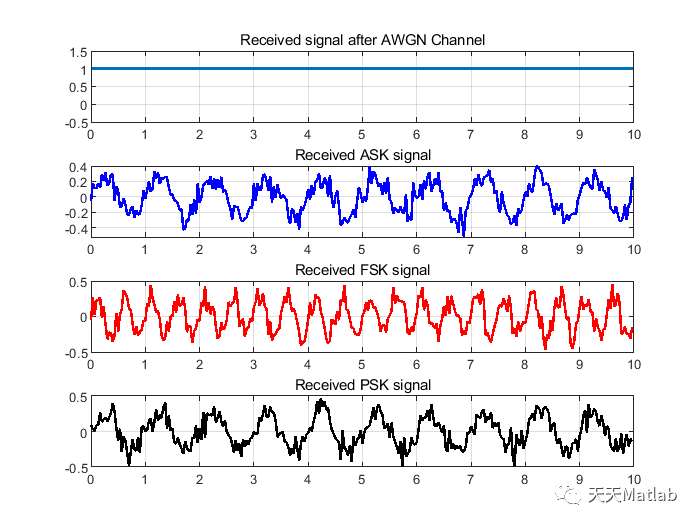0 0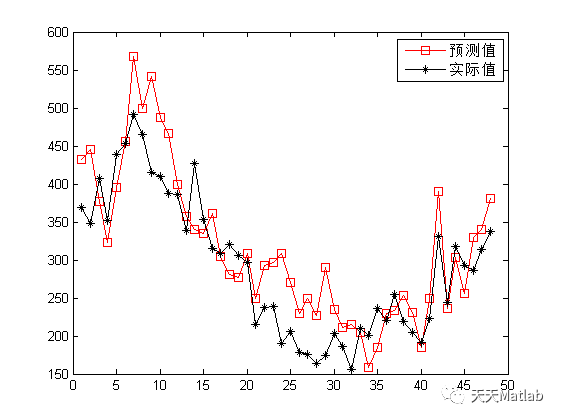【ELM回归预测】基于极限学习机实现气象影响因子光伏出力预测附matlab代码
【ELM回归预测】基于极限学习机实现气象影响因子光伏出力预测附matlab代码
0 0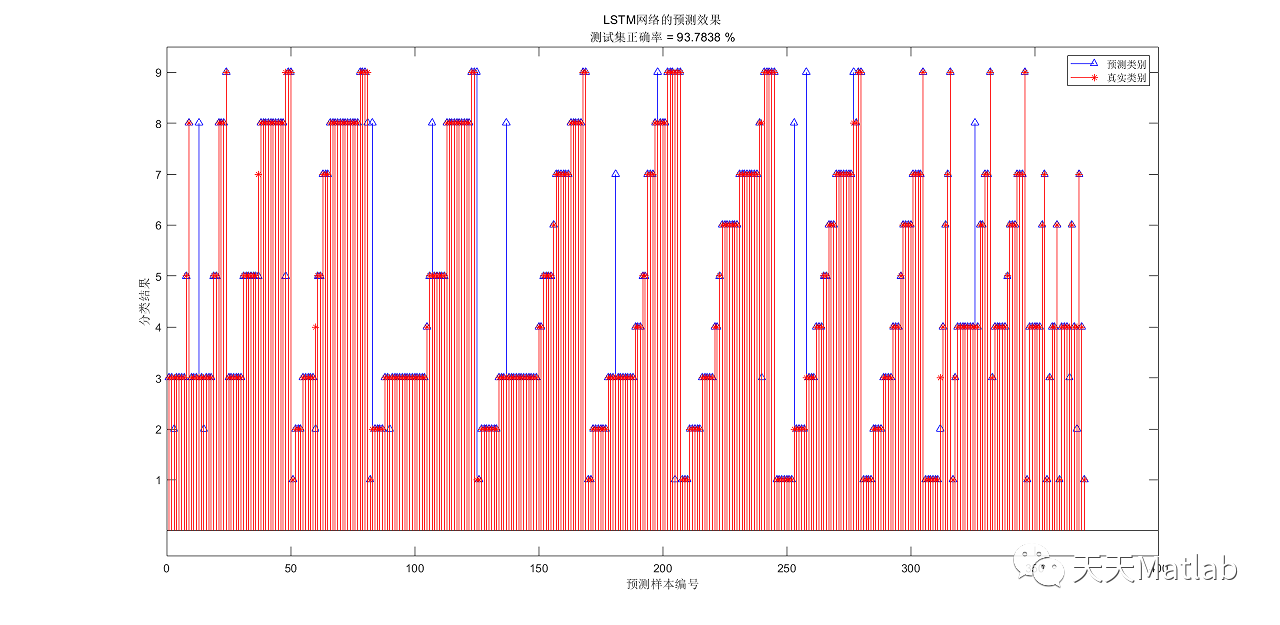0 0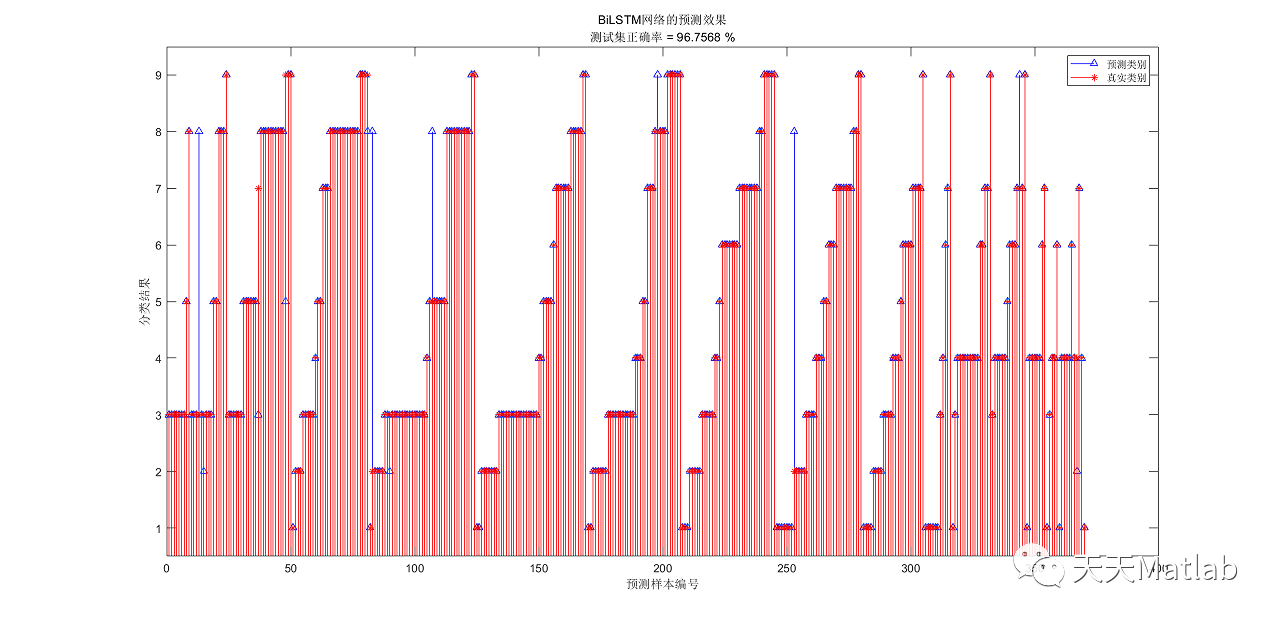0 0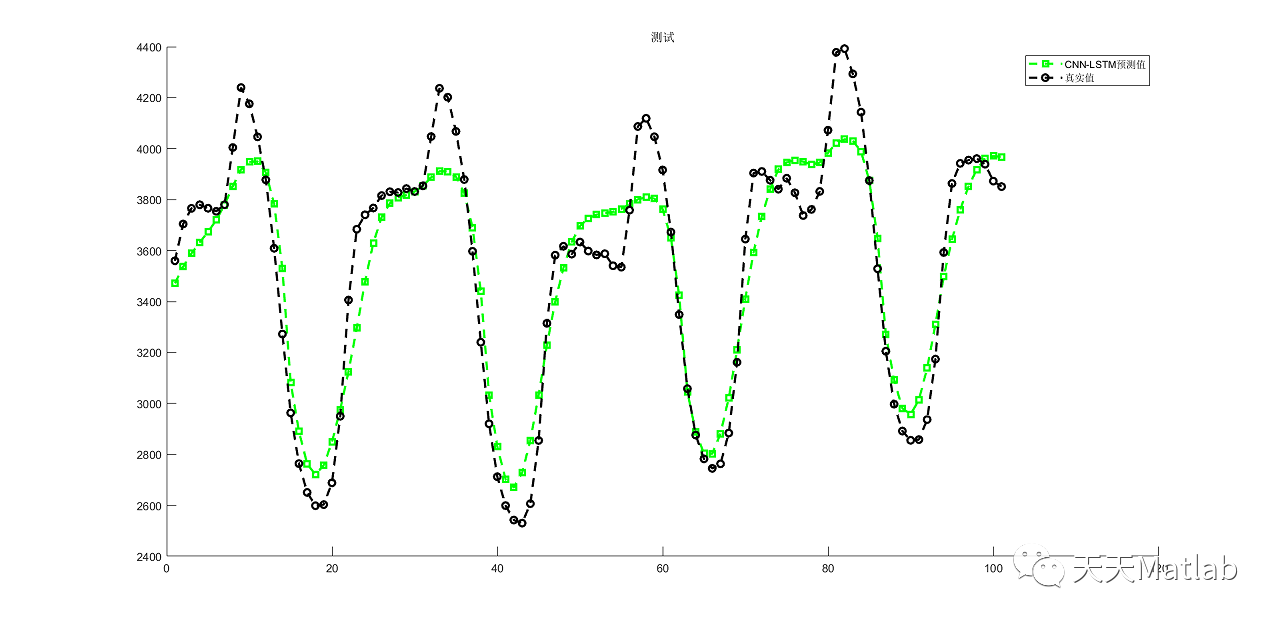【LSTM时序预测】基于卷积神经网络结合长短时记忆CNN-LSTM实现时序数据预测附matlab代码
【LSTM时序预测】基于卷积神经网络结合长短时记忆CNN-LSTM实现时序数据预测附matlab代码
0 0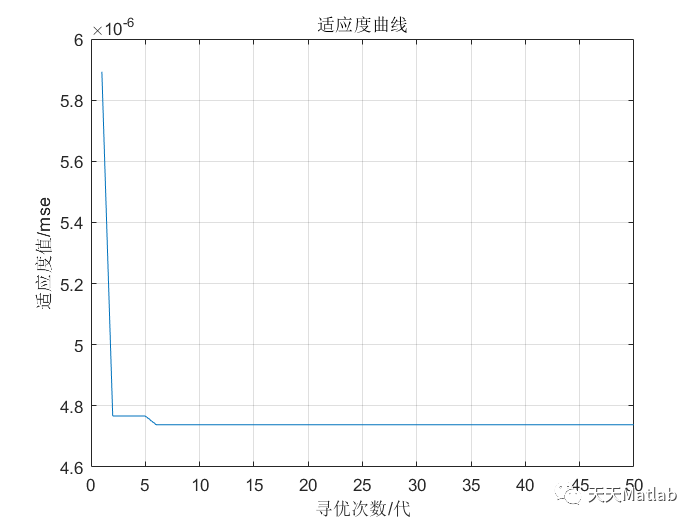【Kelm回归预测】基于粒子群算法优化核极限学习机实现数据回归预测附matlab代码
【Kelm回归预测】基于粒子群算法优化核极限学习机实现数据回归预测附matlab代码
0 0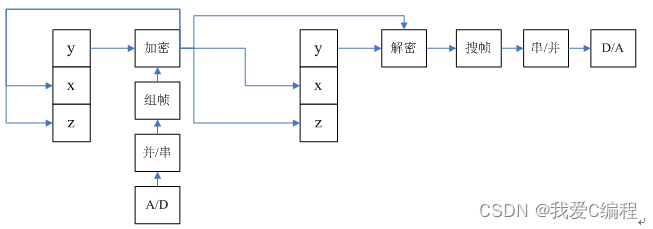m基于Lorenz混沌自同步的混沌数字保密通信系统的FPGA实现,verilog编程实现+MATLAB混沌验证程序
m基于Lorenz混沌自同步的混沌数字保密通信系统的FPGA实现,verilog编程实现+MATLAB混沌验证程序
0 0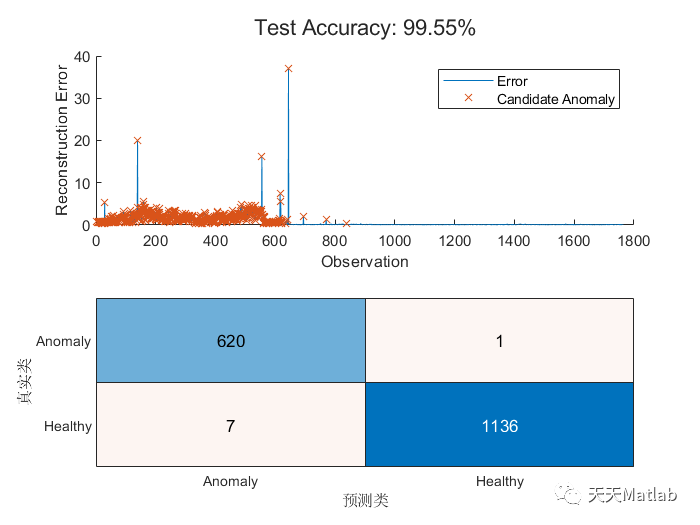【信号检测】基于LSTM实现工业机器信号数据异常检测附matlab代码
【信号检测】基于LSTM实现工业机器信号数据异常检测附matlab代码
0 0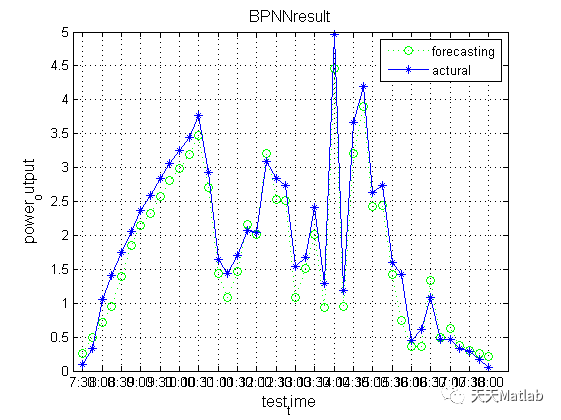【BP回归预测】基于遗传算法优化BP神经网络GA-BP实现光伏出力预测附matlab代码
【BP回归预测】基于遗传算法优化BP神经网络GA-BP实现光伏出力预测附matlab代码
0 0
Matlab科研工作室